Author: Oscar Cronquist Article last updated on April 20, 2018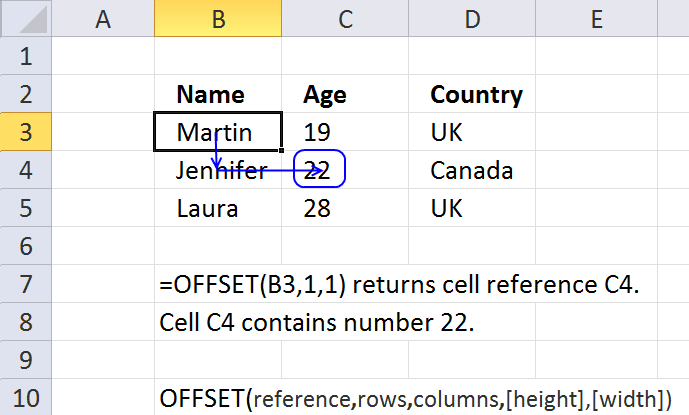The OFFSET function returns a reference to a range that is a given number of rows and columns from a given reference.

### Excel Function Syntax

OFFSET(reference,rows,columns,[height],[width])

### Arguments

 reference Required. A cell reference from which you want to start. rows Required. The number of rows you want to move from the start cell. The number can be positive or negative. columns Required. The number of columns you want to move from the start cell. This argument can also be a positive or negative number. [height] Optional. The number of rows you want to include to the returned reference. Must be a positive number. [width] Optional. The number of columns you want to include to the returned reference. Must be a positive number.

If [height] and [width] arguments are not used OFFSET function returns a cell reference with the same number of rows and columns as argument reference.

The OFFSET function returns #REF error if the returning cell reference is outside the cell grid.

### Examples

In this post I am going to provide some basic examples to demonstrate how the OFFSET function works. I also show practical examples where I use the OFFSET function. To be honest, in most cases I prefer using the INDEX function. The offset functions is volatile and extensive use in a workbook may slow down calculations noticeably.

### Example 1 - Basic demonstration

This example uses only the two first arguments in the OFFSET function, row and column.B3 is the start cell. 1 row below B3 is B4. 1 column to the right of B4 is C4.

The OFFSET function returns a cell reference to cell C4. Cell C4 contains number 22.

### Example 2 - Row function and offset function

This example shows that the offset function returns a cell reference.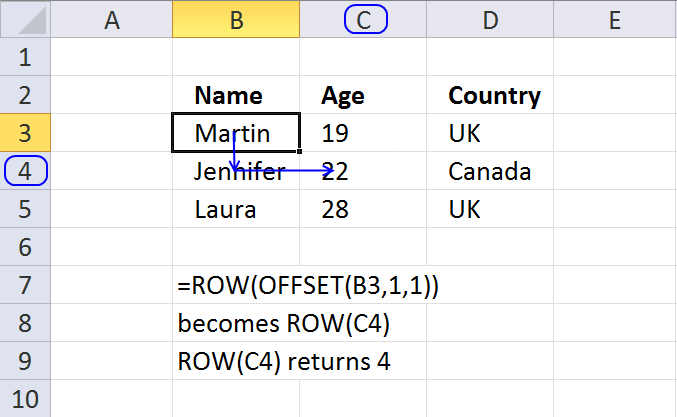ROW(reference) returns the row number of a reference. Combining the ROW and OFFSET functions demonstrates that the OFFSET function returns a cell reference.

### Example 3 - Explaining row and height arguments. OFFSET function returns a reference to a cell range

The Offset function can also return a reference to a cell range. For this to work you need to enter the formula as an array formula.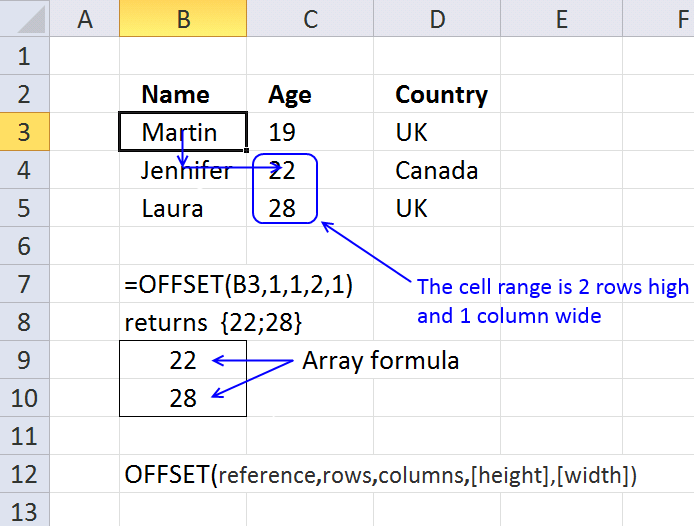Array formula in cell range B9:B10:

=OFFSET(B3,1,1,2,1)

The optional arguments height and width are used in this exampe. The height is 2 and the width is 1, that is 2 cells high and 1 cell wide.

The array formula returns a reference to a cell range with the same width and height as the two last arguments.

### How to enter an array formula

1. Select cell range B9:B10
2. Click in formula bar3. Paste array formula to formula bar
4. Press and hold CTRL + SHIFT
5. Press Enter
6. Release all keys

### Example 4 - SUBTOTAL and OFFSET functions

This example shows how to determine if a row in an excel defined table is hidden or visible.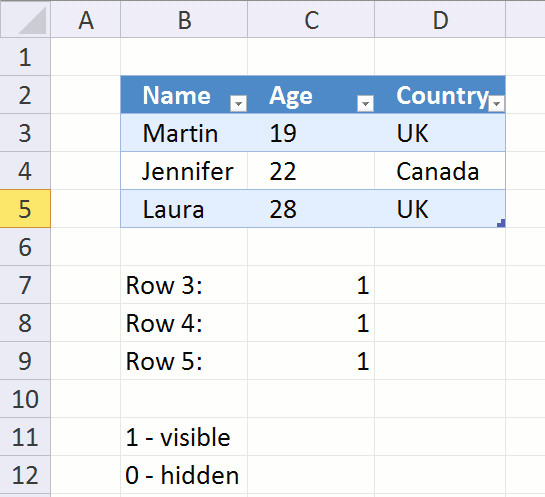Array formula in cell range C7:C9:

=SUBTOTAL(3,OFFSET(B3,MATCH(ROW(Table1[Name]),ROW(Table1[Name]))-1,0,1))

The technique is described here by David Hager and John Walkenbach: Excel Experts E-letter

I have written a couple of posts and developed this technique one step further:

### Example 5 - Named ranges and the OFFSET function

You can use the OFFSET function to create a dynamic named range. First I want to explain what named ranges is and how you can use them.

So what is a named range? You can select a cell or a range and name it. When you enter the arguments in a function you can use the name instead of the corresponding cell reference. Why? This makes it easier to read and understand a function. Compare this function =Sum(C1:C11) to this =Sum(Sales2011). The latter is easier to understand.

What is dynamic named range? It is a cell range that expands automatically when new values are added.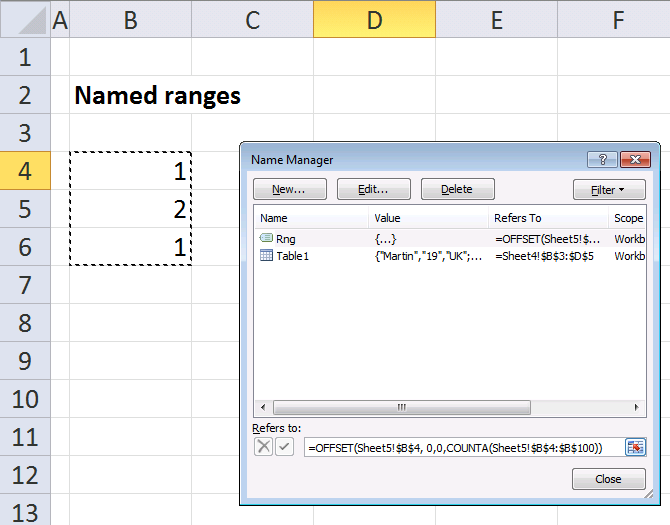Named range formula:

=OFFSET(Sheet5!\$B\$4, 0,0,COUNTA(Sheet5!\$B\$4:\$B\$100))

COUNTA counts the number of cells in a range that are not empty. COUNTA(Sheet5!\$B\$4:\$B\$100) returns 3.

=OFFSET(Sheet5!\$B\$4, 0,0,COUNTA(Sheet5!\$B\$4:\$B\$100))

becomes

=OFFSET(Sheet5!\$B\$4, 0,0,3)

and returns a reference to cell range B4:B6.